Anzeige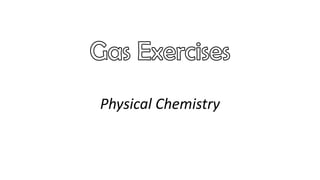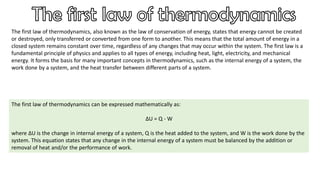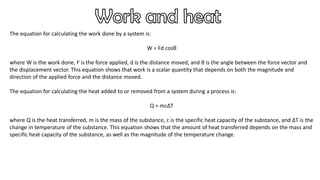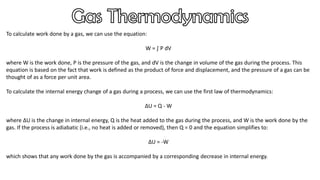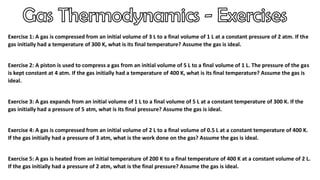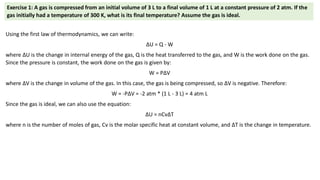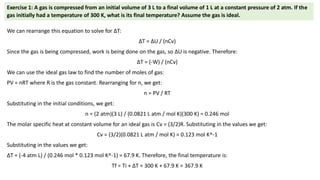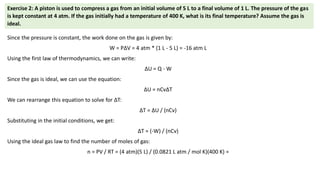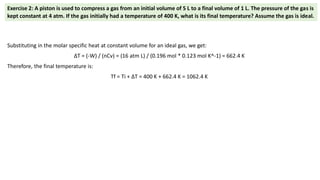1 von 9
Anzeige

### Gas Exercises.pdf

1. Physical Chemistry
2. The first law of thermodynamics, also known as the law of conservation of energy, states that energy cannot be created or destroyed, only transferred or converted from one form to another. This means that the total amount of energy in a closed system remains constant over time, regardless of any changes that may occur within the system. The first law is a fundamental principle of physics and applies to all types of energy, including heat, light, electricity, and mechanical energy. It forms the basis for many important concepts in thermodynamics, such as the internal energy of a system, the work done by a system, and the heat transfer between different parts of a system. The first law of thermodynamics can be expressed mathematically as: ΔU = Q - W where ΔU is the change in internal energy of a system, Q is the heat added to the system, and W is the work done by the system. This equation states that any change in the internal energy of a system must be balanced by the addition or removal of heat and/or the performance of work.
3. The equation for calculating the work done by a system is: W = Fd cosθ where W is the work done, F is the force applied, d is the distance moved, and θ is the angle between the force vector and the displacement vector. This equation shows that work is a scalar quantity that depends on both the magnitude and direction of the applied force and the distance moved. The equation for calculating the heat added to or removed from a system during a process is: Q = mcΔT where Q is the heat transferred, m is the mass of the substance, c is the specific heat capacity of the substance, and ΔT is the change in temperature of the substance. This equation shows that the amount of heat transferred depends on the mass and specific heat capacity of the substance, as well as the magnitude of the temperature change.
4. To calculate work done by a gas, we can use the equation: W = ∫ P dV where W is the work done, P is the pressure of the gas, and dV is the change in volume of the gas during the process. This equation is based on the fact that work is defined as the product of force and displacement, and the pressure of a gas can be thought of as a force per unit area. To calculate the internal energy change of a gas during a process, we can use the first law of thermodynamics: ΔU = Q - W where ΔU is the change in internal energy, Q is the heat added to the gas during the process, and W is the work done by the gas. If the process is adiabatic (i.e., no heat is added or removed), then Q = 0 and the equation simplifies to: ΔU = -W which shows that any work done by the gas is accompanied by a corresponding decrease in internal energy.
5. Exercise 1: A gas is compressed from an initial volume of 3 L to a final volume of 1 L at a constant pressure of 2 atm. If the gas initially had a temperature of 300 K, what is its final temperature? Assume the gas is ideal. Exercise 2: A piston is used to compress a gas from an initial volume of 5 L to a final volume of 1 L. The pressure of the gas is kept constant at 4 atm. If the gas initially had a temperature of 400 K, what is its final temperature? Assume the gas is ideal. Exercise 3: A gas expands from an initial volume of 1 L to a final volume of 5 L at a constant temperature of 300 K. If the gas initially had a pressure of 5 atm, what is its final pressure? Assume the gas is ideal. Exercise 4: A gas is compressed from an initial volume of 2 L to a final volume of 0.5 L at a constant temperature of 400 K. If the gas initially had a pressure of 3 atm, what is the work done on the gas? Assume the gas is ideal. Exercise 5: A gas is heated from an initial temperature of 200 K to a final temperature of 400 K at a constant volume of 2 L. If the gas initially had a pressure of 2 atm, what is the final pressure? Assume the gas is ideal.
6. Using the first law of thermodynamics, we can write: ΔU = Q - W where ΔU is the change in internal energy of the gas, Q is the heat transferred to the gas, and W is the work done on the gas. Since the pressure is constant, the work done on the gas is given by: W = PΔV where ΔV is the change in volume of the gas. In this case, the gas is being compressed, so ΔV is negative. Therefore: W = -PΔV = -2 atm * (1 L - 3 L) = 4 atm L Since the gas is ideal, we can also use the equation: ΔU = nCvΔT where n is the number of moles of gas, Cv is the molar specific heat at constant volume, and ΔT is the change in temperature. Exercise 1: A gas is compressed from an initial volume of 3 L to a final volume of 1 L at a constant pressure of 2 atm. If the gas initially had a temperature of 300 K, what is its final temperature? Assume the gas is ideal.
7. We can rearrange this equation to solve for ΔT: ΔT = ΔU / (nCv) Since the gas is being compressed, work is being done on the gas, so ΔU is negative. Therefore: ΔT = (-W) / (nCv) We can use the ideal gas law to find the number of moles of gas: PV = nRT where R is the gas constant. Rearranging for n, we get: n = PV / RT Substituting in the initial conditions, we get: n = (2 atm)(3 L) / (0.0821 L atm / mol K)(300 K) = 0.246 mol The molar specific heat at constant volume for an ideal gas is Cv = (3/2)R. Substituting in the values we get: Cv = (3/2)(0.0821 L atm / mol K) = 0.123 mol K^-1 Substituting in the values we get: ΔT = (-4 atm L) / (0.246 mol * 0.123 mol K^-1) = 67.9 K. Therefore, the final temperature is: Tf = Ti + ΔT = 300 K + 67.9 K = 367.9 K Exercise 1: A gas is compressed from an initial volume of 3 L to a final volume of 1 L at a constant pressure of 2 atm. If the gas initially had a temperature of 300 K, what is its final temperature? Assume the gas is ideal.
8. Exercise 2: A piston is used to compress a gas from an initial volume of 5 L to a final volume of 1 L. The pressure of the gas is kept constant at 4 atm. If the gas initially had a temperature of 400 K, what is its final temperature? Assume the gas is ideal. Since the pressure is constant, the work done on the gas is given by: W = PΔV = 4 atm * (1 L - 5 L) = -16 atm L Using the first law of thermodynamics, we can write: ΔU = Q - W Since the gas is ideal, we can use the equation: ΔU = nCvΔT We can rearrange this equation to solve for ΔT: ΔT = ΔU / (nCv) Substituting in the initial conditions, we get: ΔT = (-W) / (nCv) Using the ideal gas law to find the number of moles of gas: n = PV / RT = (4 atm)(5 L) / (0.0821 L atm / mol K)(400 K) =
9. Exercise 2: A piston is used to compress a gas from an initial volume of 5 L to a final volume of 1 L. The pressure of the gas is kept constant at 4 atm. If the gas initially had a temperature of 400 K, what is its final temperature? Assume the gas is ideal. Substituting in the molar specific heat at constant volume for an ideal gas, we get: ΔT = (-W) / (nCv) = (16 atm L) / (0.196 mol * 0.123 mol K^-1) = 662.4 K Therefore, the final temperature is: Tf = Ti + ΔT = 400 K + 662.4 K = 1062.4 K
Anzeige